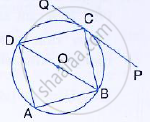Share

# In the given figure, ABCD is a cyclic quadrilateral, PQ is tangent to the circle at point C and BD is its diameter. If ∠DCQ = 40° and ∠ABD = 60°, find; (i) ∠DBC (ii) ∠BCP (iii) ∠ADB - Mathematics

Course

#### Question

In the given figure, ABCD is a cyclic quadrilateral, PQ is tangent to the circle at point C and BD is its diameter. If ∠DCQ = 40° and ∠ABD = 60°, find;

(i) ∠DBC (ii) ∠BCP (iii) ∠ADB#### Solution

(i)PQ is tangent and CD is a chord
∴ ∠DCQ = ∠ DBC (angles in the alternate segment)

DBC = 40° (∵  ∠DCQ = 40°)

ii)  ∠DCQ +  ∠DCB +  ∠BCP = 180°

⇒ 40° + 90° +  ∠BCP = 180° (∵ ∠DCB = 90°)

⇒ ∠BCP = 180° = 130° = 50°

iii) In Δ ABD

∠ADB = 180° , ∠ABD = 60°

∴  ∠ADB = 180° - ( 90° + 60°)

⇒ ∠ADB = 180° - 150° = 30°

Is there an error in this question or solution?